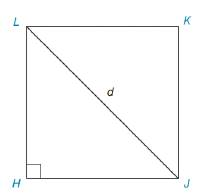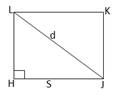Chapter 8.1, Problem 35EElementary Geometry For College St...

7th Edition
Alexander + 2 others
ISBN: 9781337614085

Solutions

Chapter
SectionElementary Geometry For College St...

7th Edition
Alexander + 2 others
ISBN: 9781337614085
Textbook Problem

In Exercises 34 to 36, provide paragraph proofs. Given: Square HJKL with L J = d Prove: A H J K L = d 2 2To determine

To prove:

AHJKL=d22 in square HKJL with LJ=d.Explanation

Given,

Length of the diagonal of square =d

(i.e.) LJ=d

Let s be the length of each side of the square.

Area of the square with length of sis(A)=s2

By right triangle ΔLHJ,

(LH)2+(HJ)2=(LJ)2

s2+s2=

Still sussing out bartleby?

Check out a sample textbook solution.

See a sample solution

The Solution to Your Study Problems

Bartleby provides explanations to thousands of textbook problems written by our experts, many with advanced degrees!

Get Started

Expand each expression in Exercises 122. (y+3)(y+4)

Finite Mathematics and Applied Calculus (MindTap Course List)

In Exercises 31-34, find an equation of the line that passes through the points. 34. (1, 2) and (3, 4)

Applied Calculus for the Managerial, Life, and Social Sciences: A Brief Approach

Rational Inequalities Solve the inequality. 31. (x1)2(x+1)(x+2)0

Precalculus: Mathematics for Calculus (Standalone Book)

Reduction Formula Describe what is meant by a reduction formula. Give an example.

Calculus: Early Transcendental Functions (MindTap Course List)

What rationalizing substitution should be made for x3+2x3+1dx? a) u = x b) u=x3 c) u=x3+2 d) u=x3+1

Study Guide for Stewart's Single Variable Calculus: Early Transcendentals, 8th